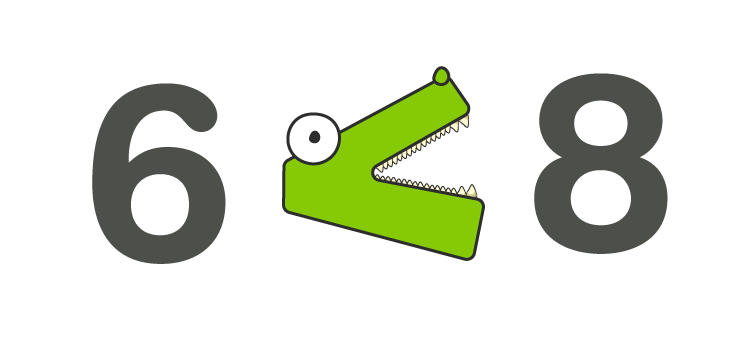# Less than Symbol

In Mathematics, the less-than symbol is a fundamental Mathematical symbol that describes the inequality between two values. There are different symbols in Maths, which are used to perform different arithmetic operations, hold some constant values, as well as used to compare the quantities with the help of equality and inequality symbols. In this article, we are going to discuss one of the inequality symbols called “Less than Symbol” in detail, with the help of many examples.

## What is Less than Symbol?

The Less-than symbol is to compare two values in which the first number is less than the second number, such as in ascending order. The less than symbol is an approximation of the opening angle bracket. The symbol used to represent the less than inequality is “< “. Less than sign is a universally adopted math symbol of two equal measure strokes that meet in the acute angle at the left.

## Less than Symbol Example

• 2 < 5: 2 is less than 5
• 0.8 < 1.2: 0.8 is less than 1.2
• -0.6 < -0. 1: -0.6 is less than -0.1

## Maths Signs

Below is a list of all the Math Signs along with less than a symbol:

 Symbol Description Example = Equal to 4+3 = 7 ≠ Not equal to 5 + 3 ≠ 9 > Greater than 8 > 6 ≥ Greater than or equal to Students ≥ 8 < Less than 6 < 8 ≤ Less than or equal to Students ≤ 70

## How to Remember the Less than Sign?

The best way to remember the less-than symbol is the “Alligator Method“. While comparing two values, think of the alligator method. It is known that the alligator’s mouth always points to the largest value, the reason being it can eat as much as possible. The alligator’s mouth always opens to the right to represent the less than inequality. We can also use the alligator method to use greater than a symbol. The alligator mouth points to the left to define the greater than symbol.

Example:

The representation of less than inequality between two numbers say 6 and 8, is written by

• 6 < 8Another method to remember the less-than sign is the “L Method”. L method is pretty easy to remember the less than a symbol. “Less than” starts with the letter “L”  and the symbol that represents the less-than sign (<) almost looks similar to the letter L.

There are some other terminologies used alternatively with less than such as:

• Smaller than
• Lower than
• Fewer than
• Not greater than
• Below

### Less than Symbol Word Problems

Before solving the less than sign inequality word problems, consider some of the strategies given below to solve the problem.

• Go through the complete problem
• Highlight the relevant and necessary keywords to resolve the problem
• Identify the variables
• Scribble down the equation
• Solve the problem
• Write the solution in comprehensive sentence format

### Solved Example

Question: Arjun’s April profit of Rs.150 was at least Rs.11 less than his March month profit. Determine Arjun’s March month profit?

Solution:

The given statement is “April profit of Rs.150 was at least Rs.11 less than his March month profit”

Let “P” be the profit decrease from March to April

So, the inequality expression is

-11+P ≤ 150

Add 11 on both sides, the inequality expression becomes,

P ≤ 161

Therefore, the March month profit of Arjun’s was almost Rs.161.

## Frequently Asked Questions on Less than Symbol

Q1

### What is the use of less than sign?

The less-than sign is one of the inequality signs, which is used to compare the number. If the first number is less than the second number, less sign is used. For example, 5 < 10. It means that the number 5 is less than the number 10.

Q2

### What are the other terms used to describe less than?

The other terms used to describe less than is smaller than, lower than, below, fewer than, not greater than, and so on.

Q3

### How to represent “9 is less than 20” symbolically?

“9 is less than 20” is symbolically represented as 9 < 20.

Q4

### What are the 2 tricks used to remember the less than sign?

The less than sign can be remembered using:
Alligator Method
L Method

Q5

### How to write 60 is not greater than 70?

60 is not greater than 70 is mathematically represented as 60 < 70.

Stay tuned with BYJU’S – The Learning App for more maths-related articles and materials. Also, watch engaging videos to learn with ease.

 Related Links Fractions Mixed Fractions Decimals Integers